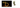# Introduction to Big O Notation## What is Big O?

In computer science, big O notation is used to analyze how their running time or the space used by an algorithm.it is invented by Paul Bachmann, Edmund Landau.

Let’s discuss some common time complexities with the help of examples.

## Constant time O(1)

If an algorithm has a constant time, it means that it always takes the same amount of time to produce the output.

Example

``````function removeLastitem(arr){
return arr.pop()
}

console.log(removeLastitem([1,2,3,4,5,6]))``````

In the above example, removeLastitem function always takes the same amount of time to remove the last item from the array it doesn’t matter if the array has 10 items or 20 items.

## Linear time O(n)

if an algorithm has a linear time, it means that the running time of an algorithm grows as the input size grows.

example

``````function sum(arr) {
let total = 0;
for (let i = 0; i < arr.length; i = i + 1) {
total += arr[i];
}
}

console.log(sum([1, 2, 3, 4])) //10``````

In the above example,sum function increases its running time according to the size of the array.

The running time of an algorithm is directly proportional to the square of the size of the input.

example :

``````function addAndLog(arr) {
for (var i = 0; i < arr.length; i++) {
for (var j = 0; j < arr.length; j++) {
console.log(arr[i] + arr[j])
}//O(n)
console.log("----")
}// O(n)
}``````

Happy coding …

## Top Udemy Courses##### JavaScript - The Complete Guide 2020 (Beginner + Advanced)
45,614 students enrolled
52 hours of video content
View Course##### React - The Complete Guide (incl Hooks, React Router, Redux)
284,472 students enrolled
40 hours of video content
View Course##### Vue - The Complete Guide (w/ Router, Vuex, Composition API)
130,921 students enrolled
48.5 hours of video content
View Course